# 直观理解贝叶斯定理

/ 0评 / 5

## 介绍

### 公式

P(H|E) = \frac{P(H)·P(E|H)}{P(E)}

## 理解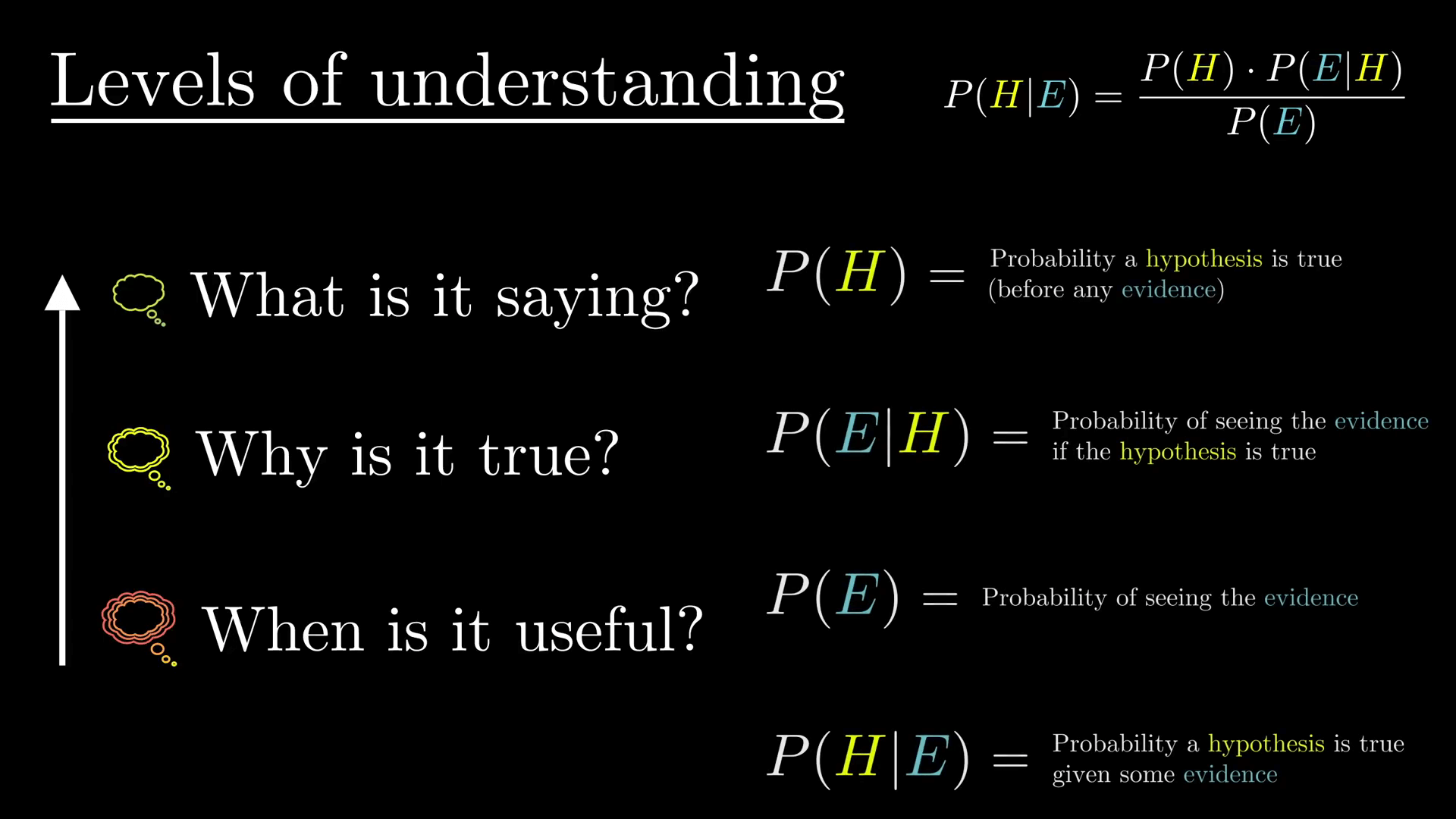## 举例一

### 描述

Steve 非常害羞而且性格孤僻，虽然总是乐于助人，但对周围的人或现实世界几乎不感兴趣，他是一个温顺而又井井有条（meek and tidy）的人，需要事情有条理且结构清晰，并乐于钻研细节。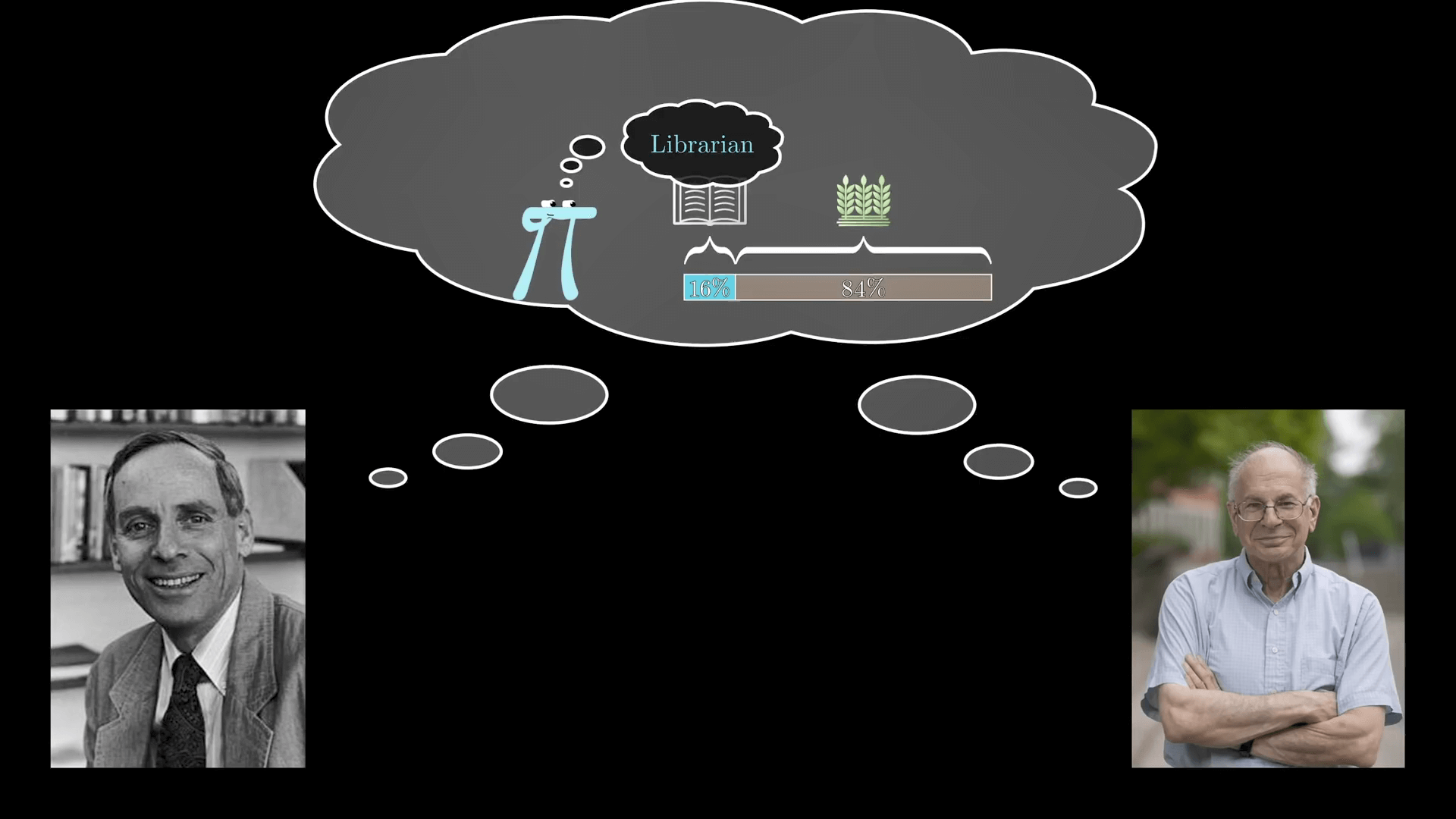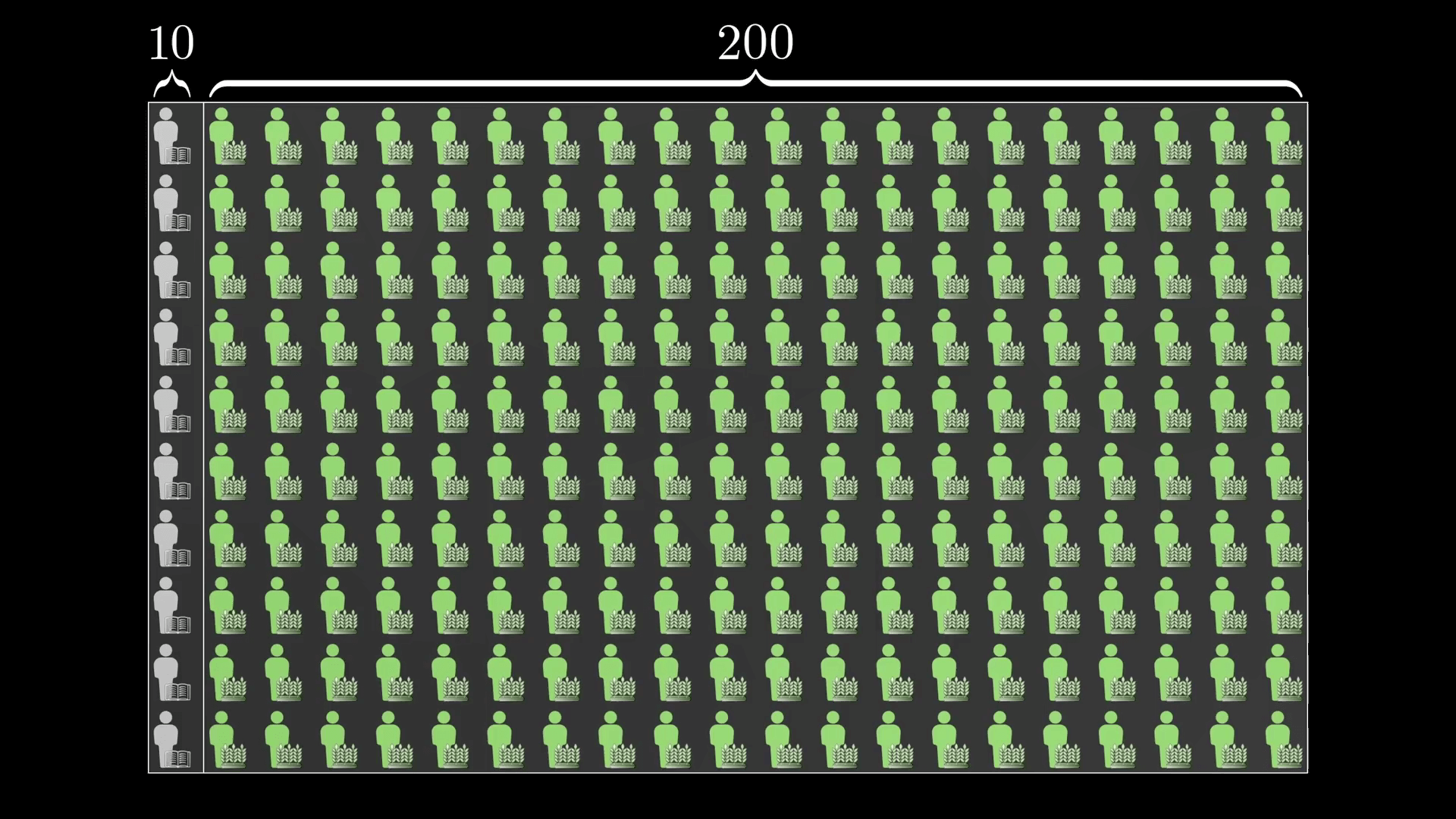### 求解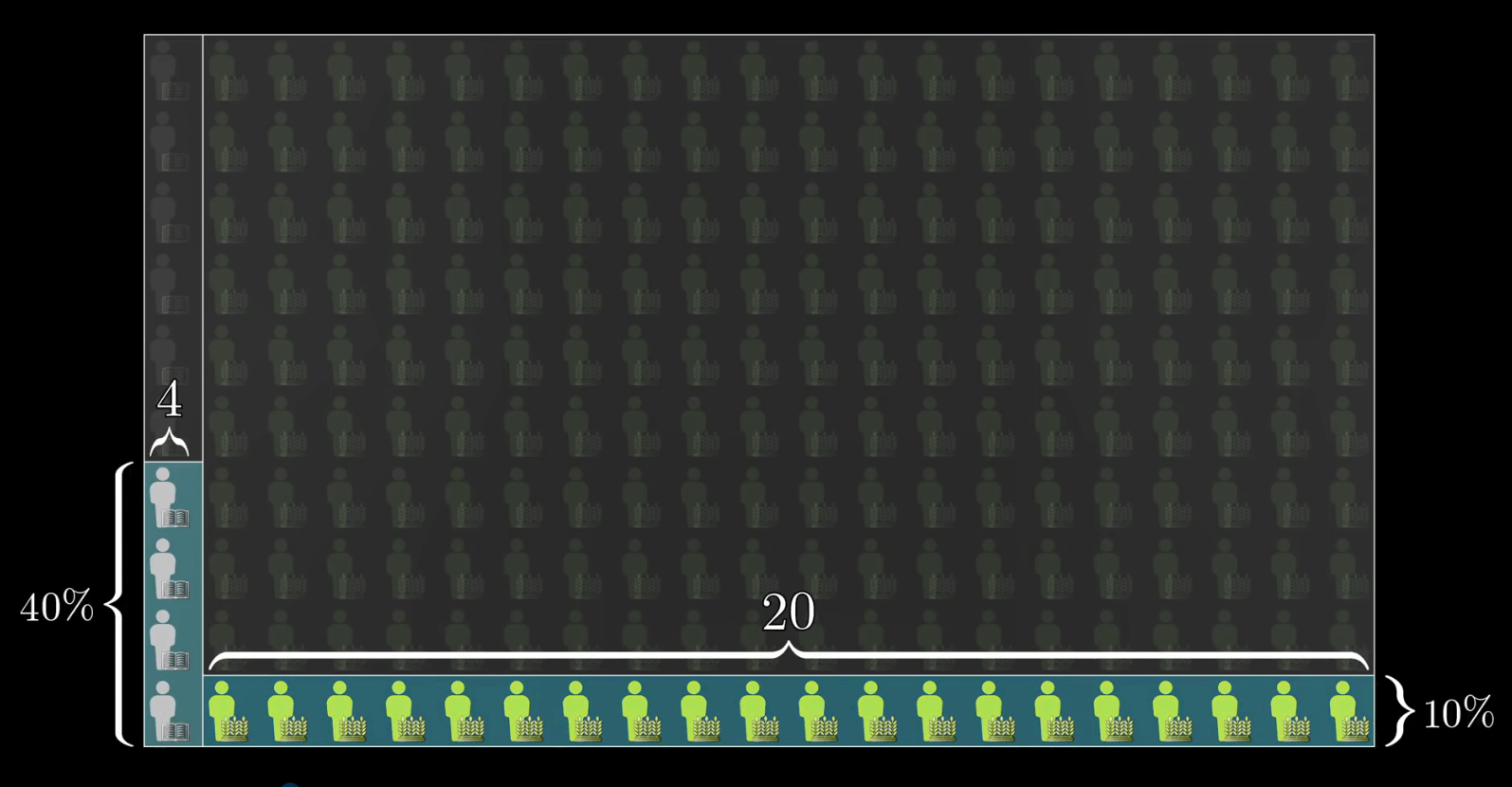P(Librarian \ and \ Description) = \frac{4}{4+20} ≈ 16.7\%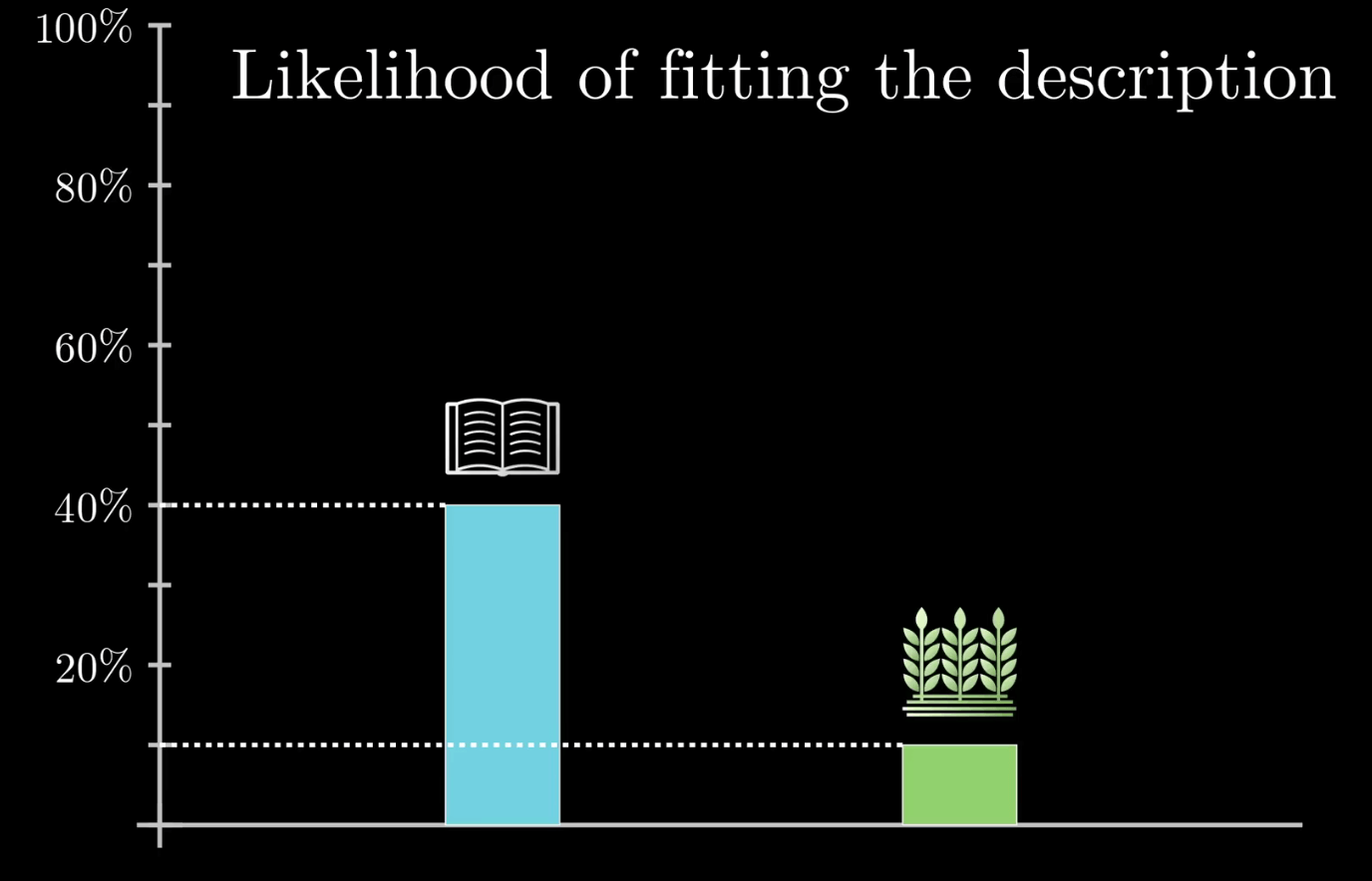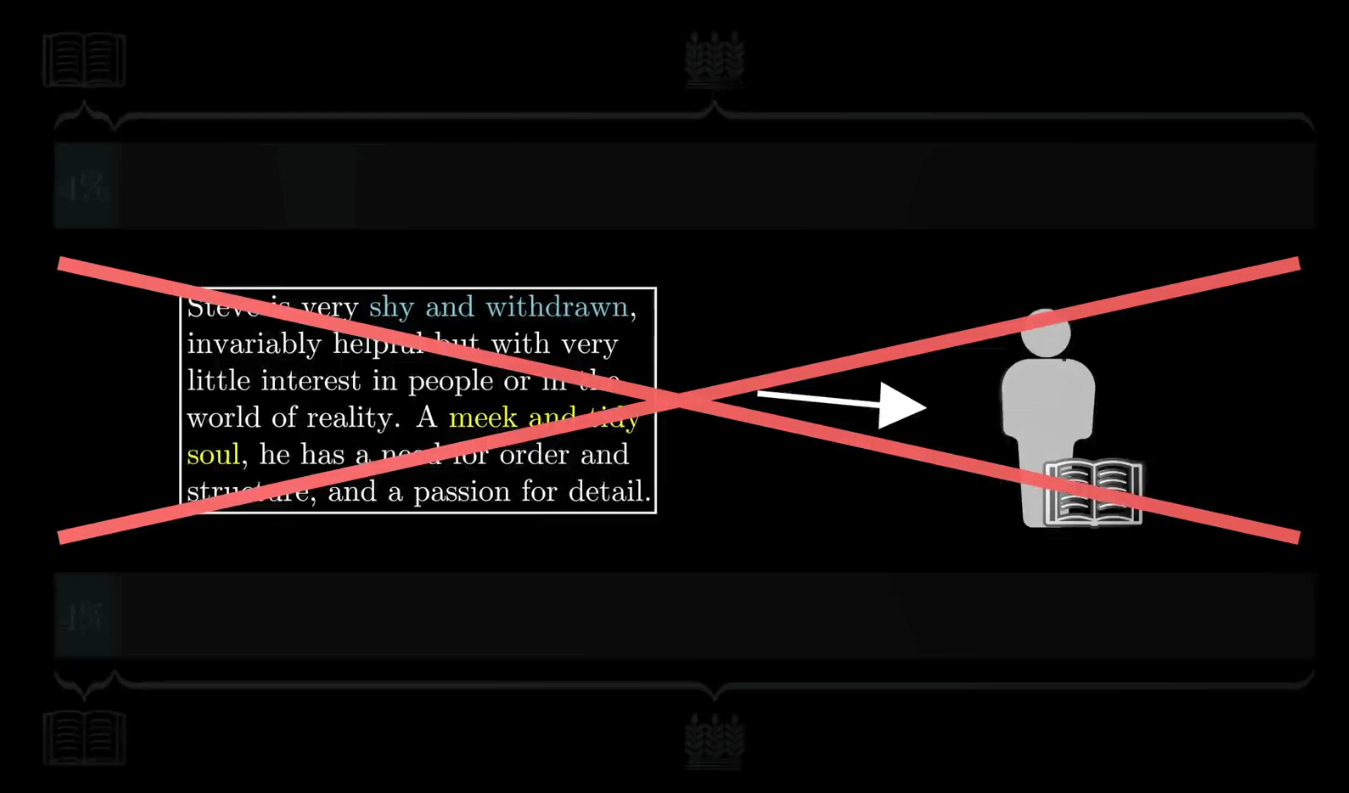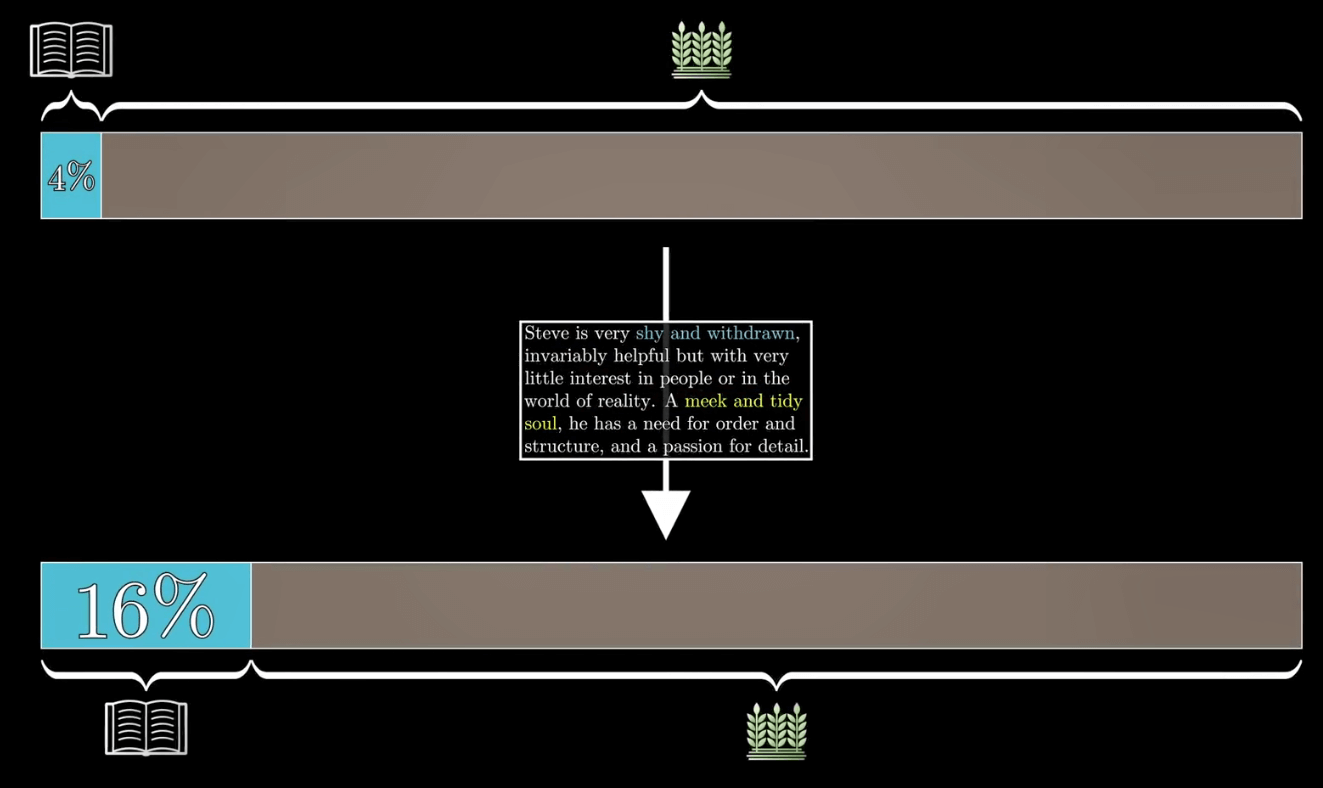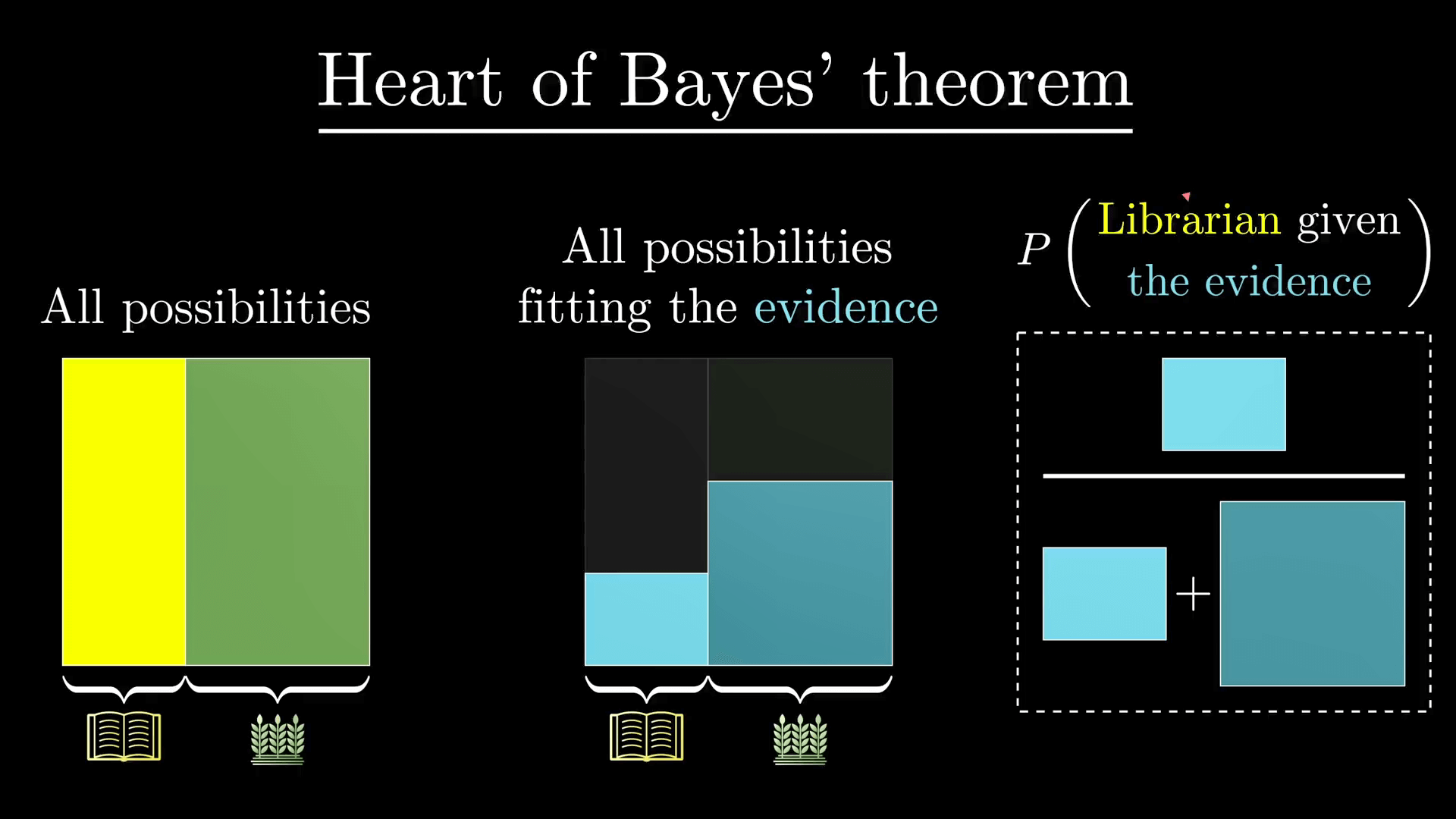## 形式化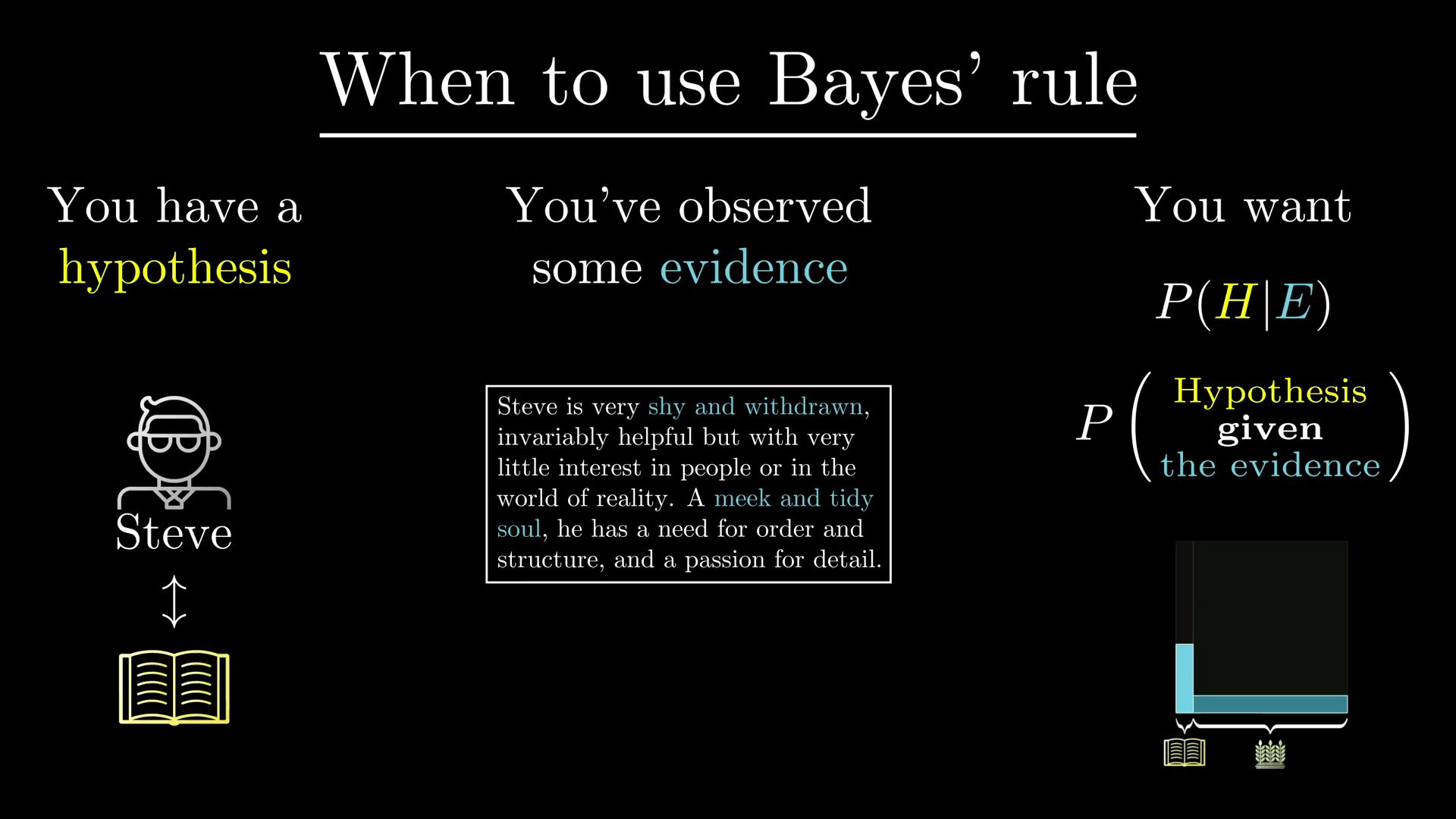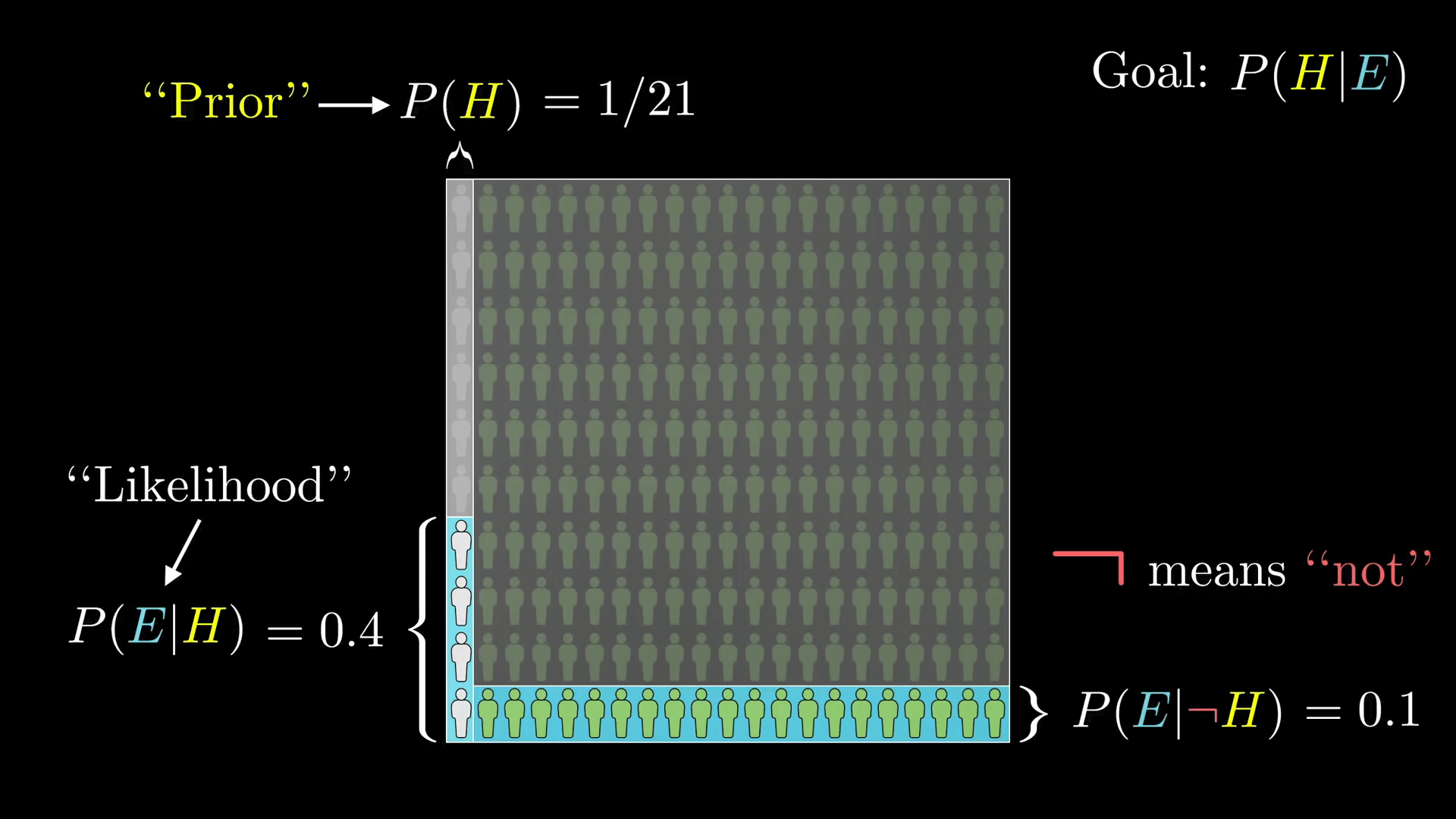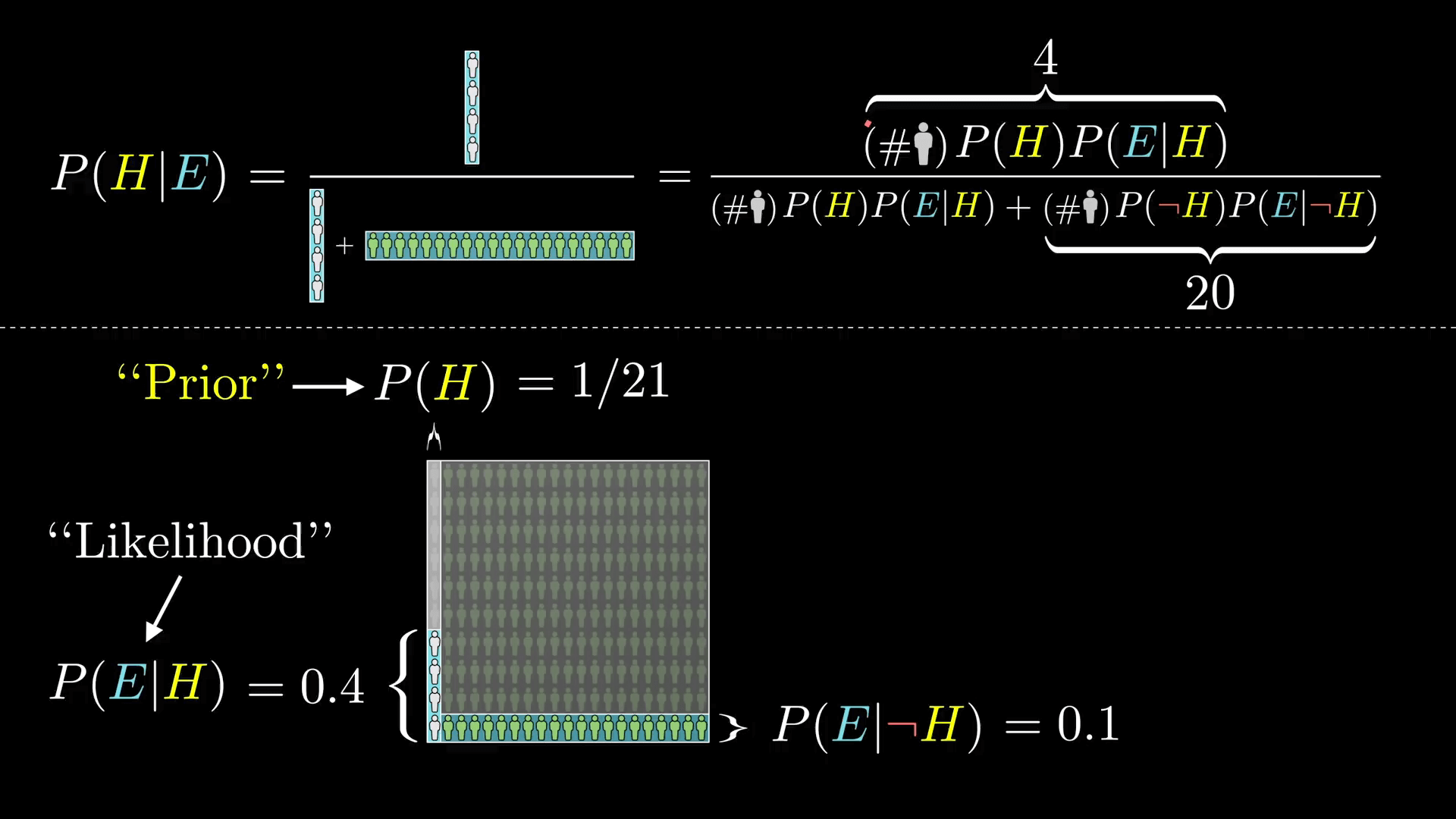P(H|E) = \frac{N·P(H)P(E|H)}{N·P(H)P(E|H) + N·P(┐H)P(E|┐H)}

P(H|E) = \frac{P(H)P(E|H)}{P(H)P(E|H) + P(┐H)P(E|┐H)}

P(H|E) = \frac{P(H)·P(E|H)}{P(E)}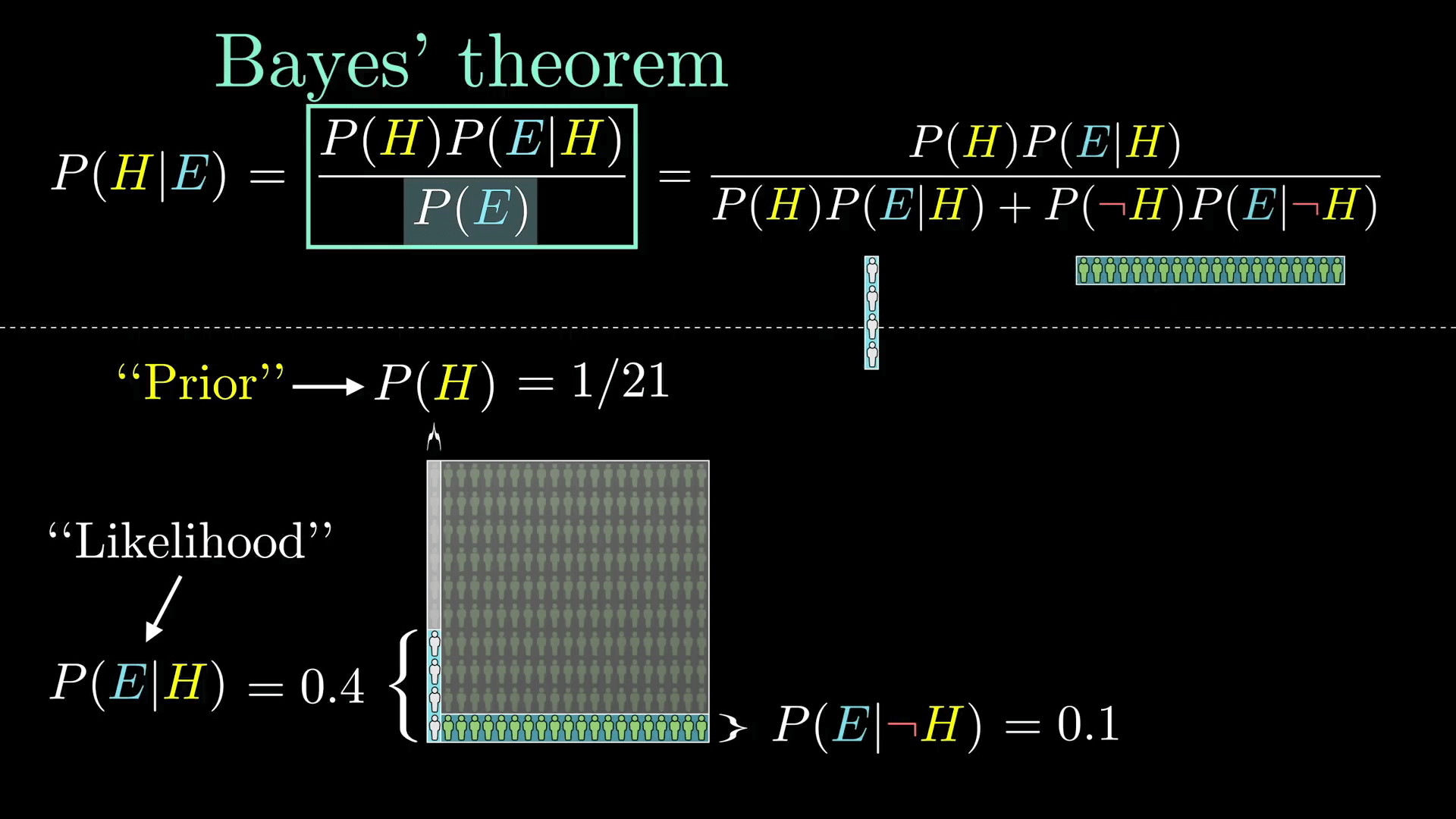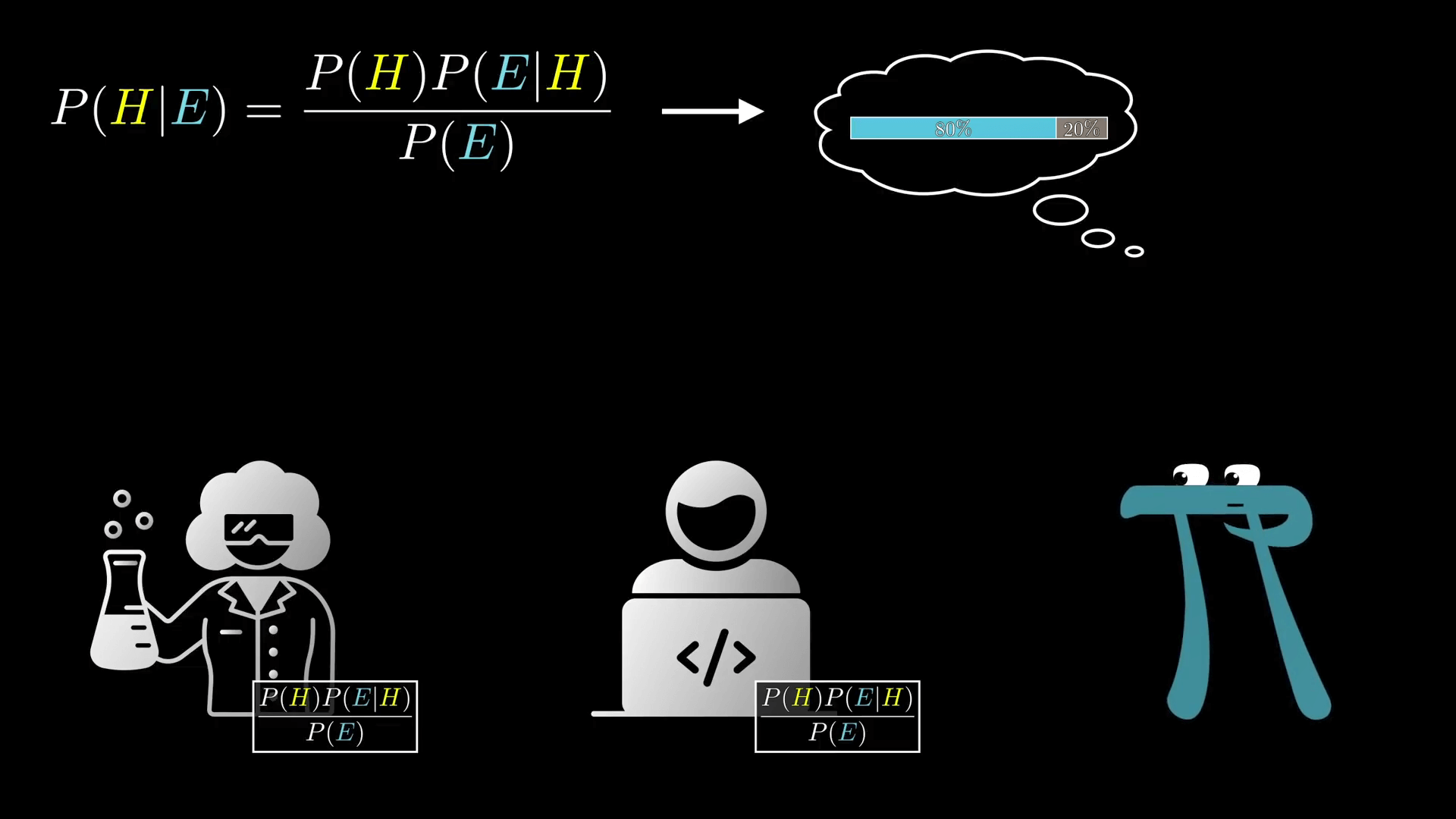## 使用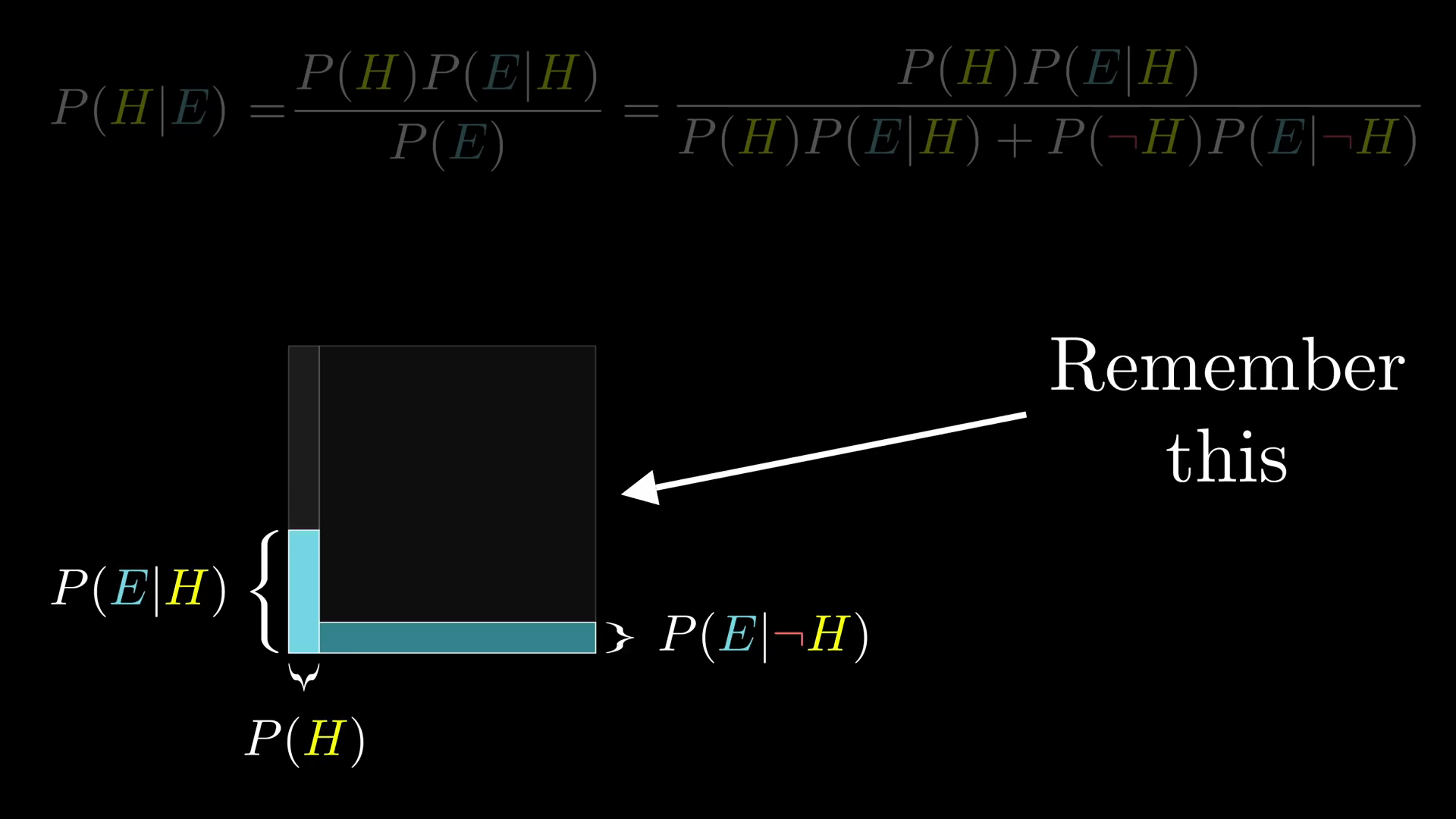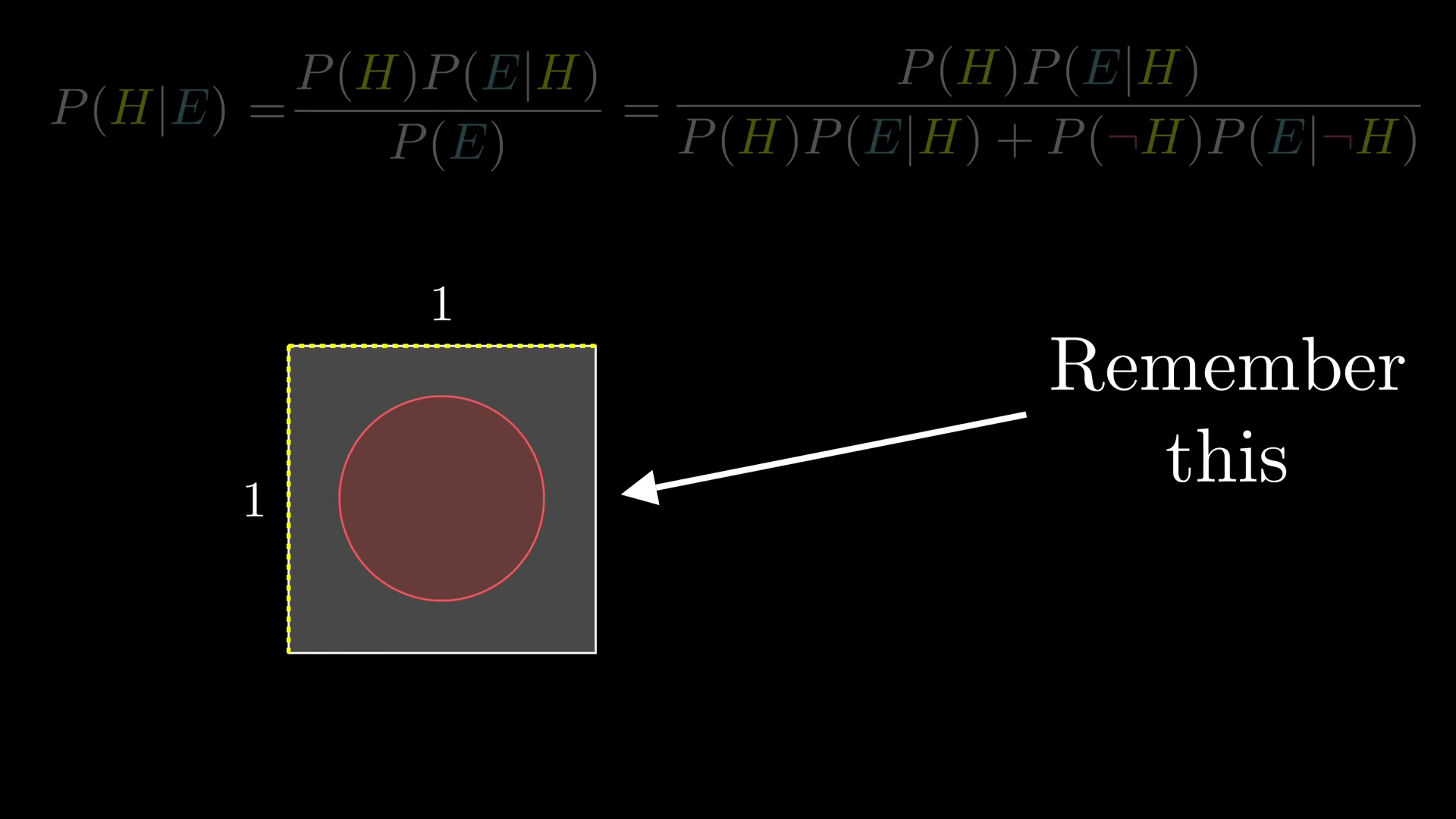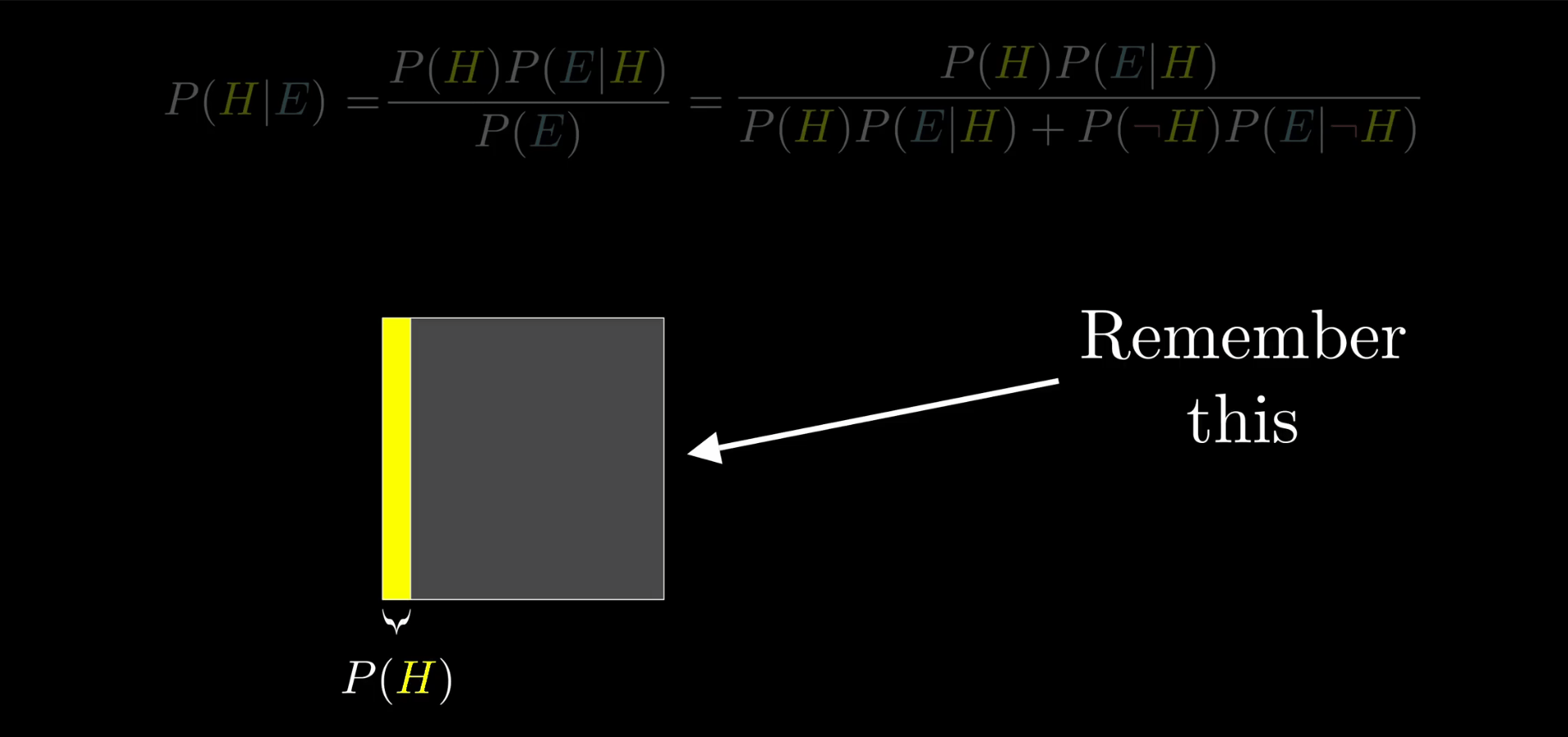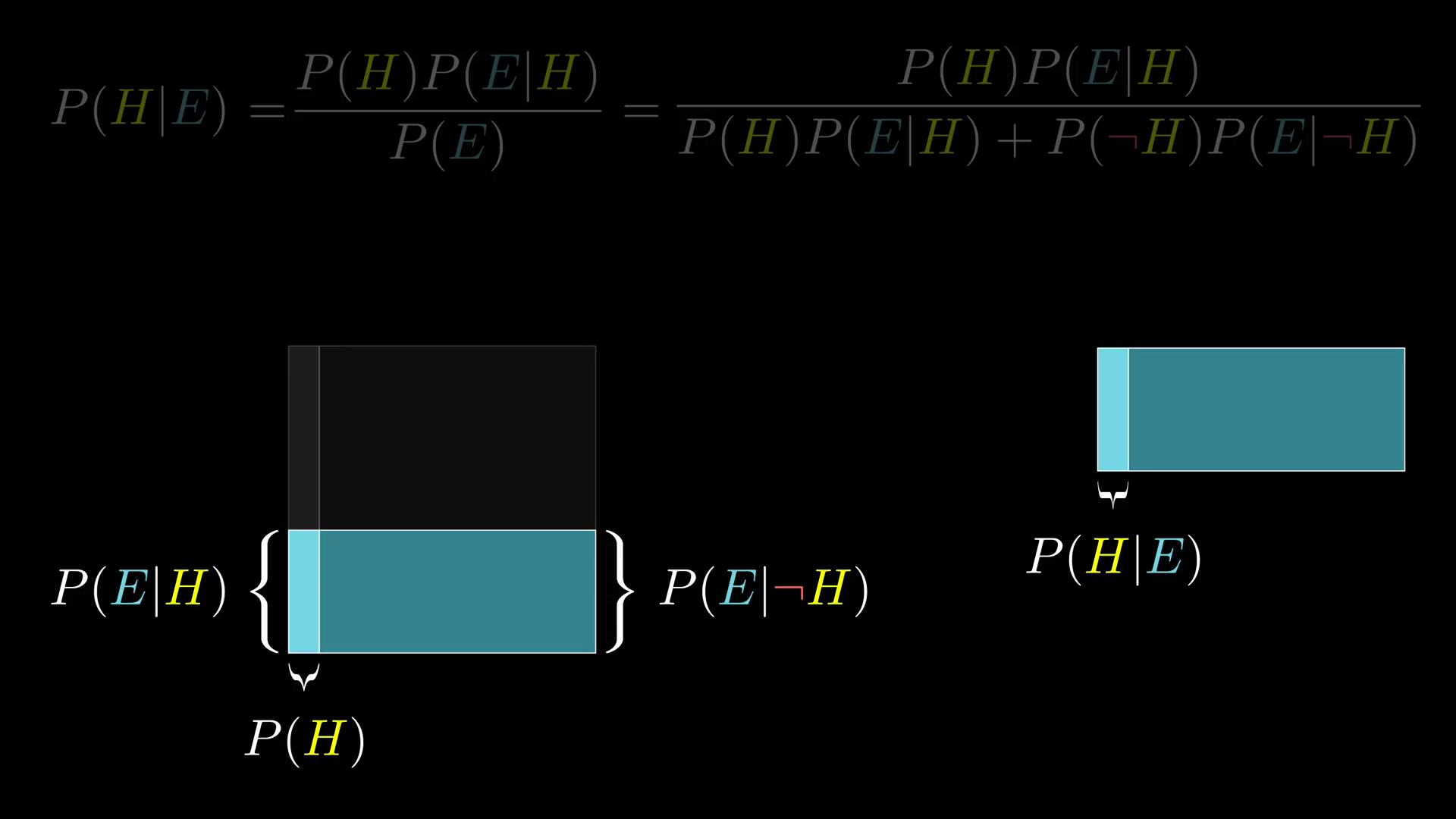## 举例二

### 描述

85% 的参与者说后者更有可能，尽管“是银行出纳员同时也是女权运动中的一个积极分子”是“银行出纳员”的一个子集，前者肯定是比后者少的。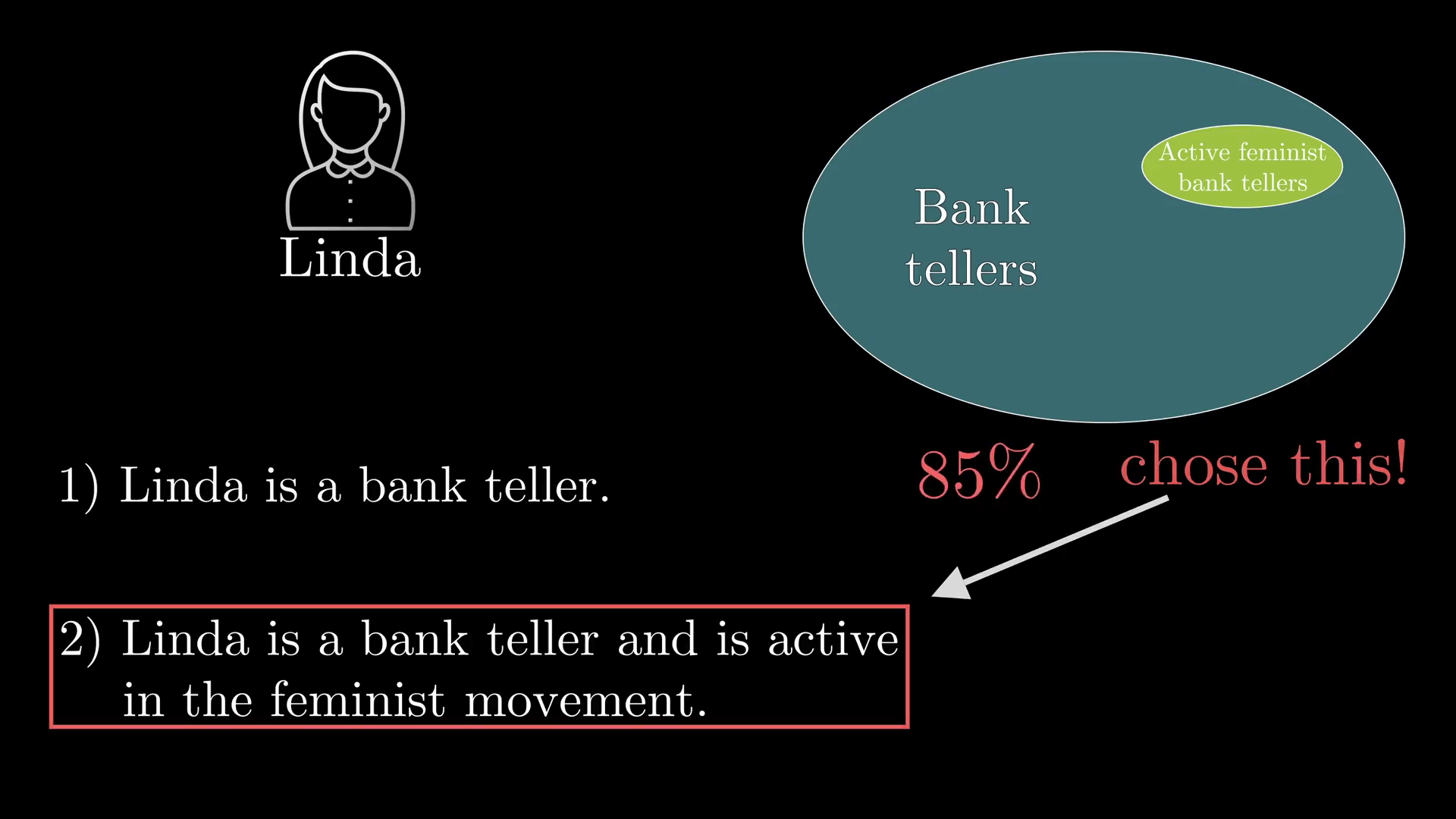### 思考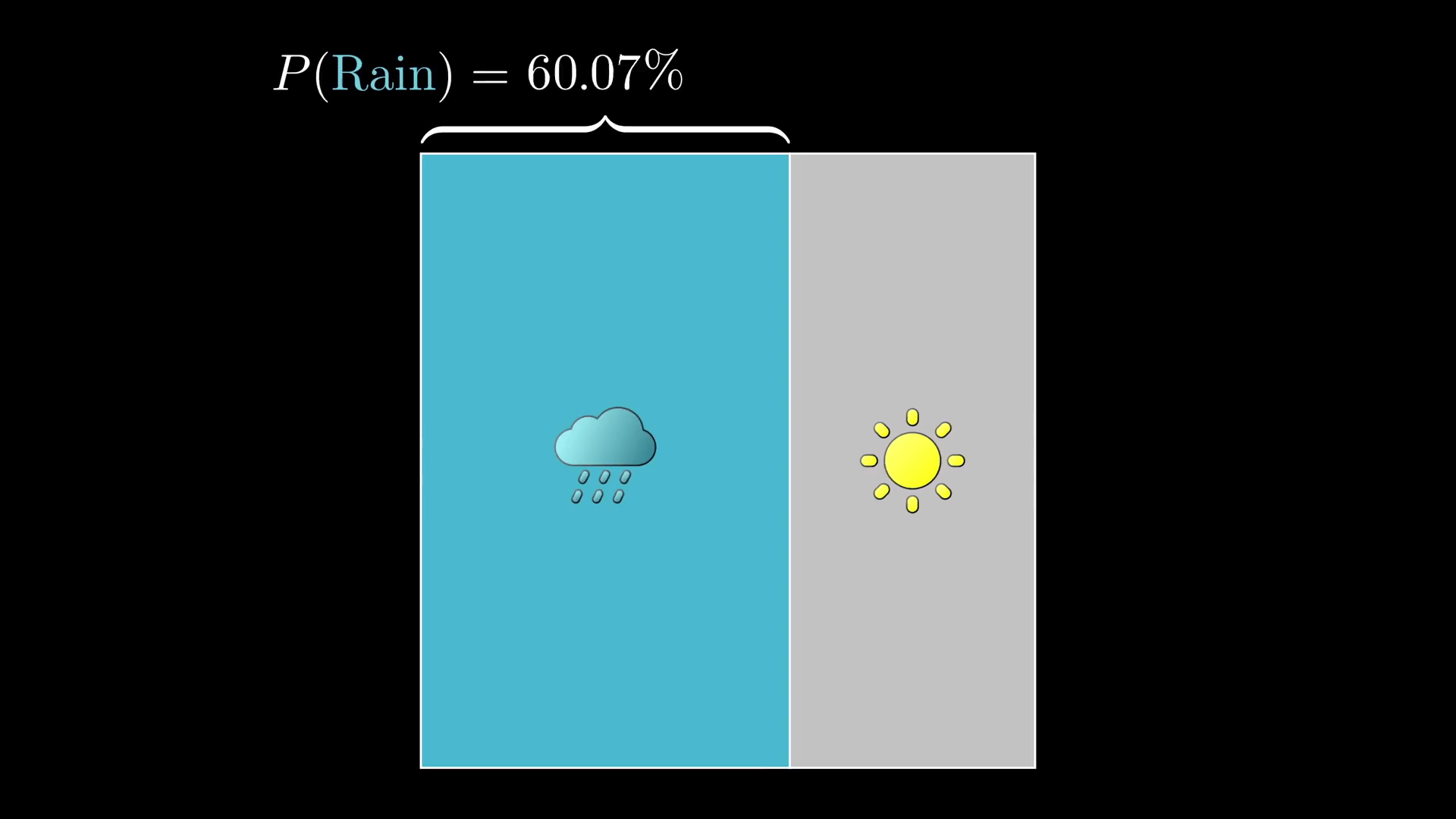## 总结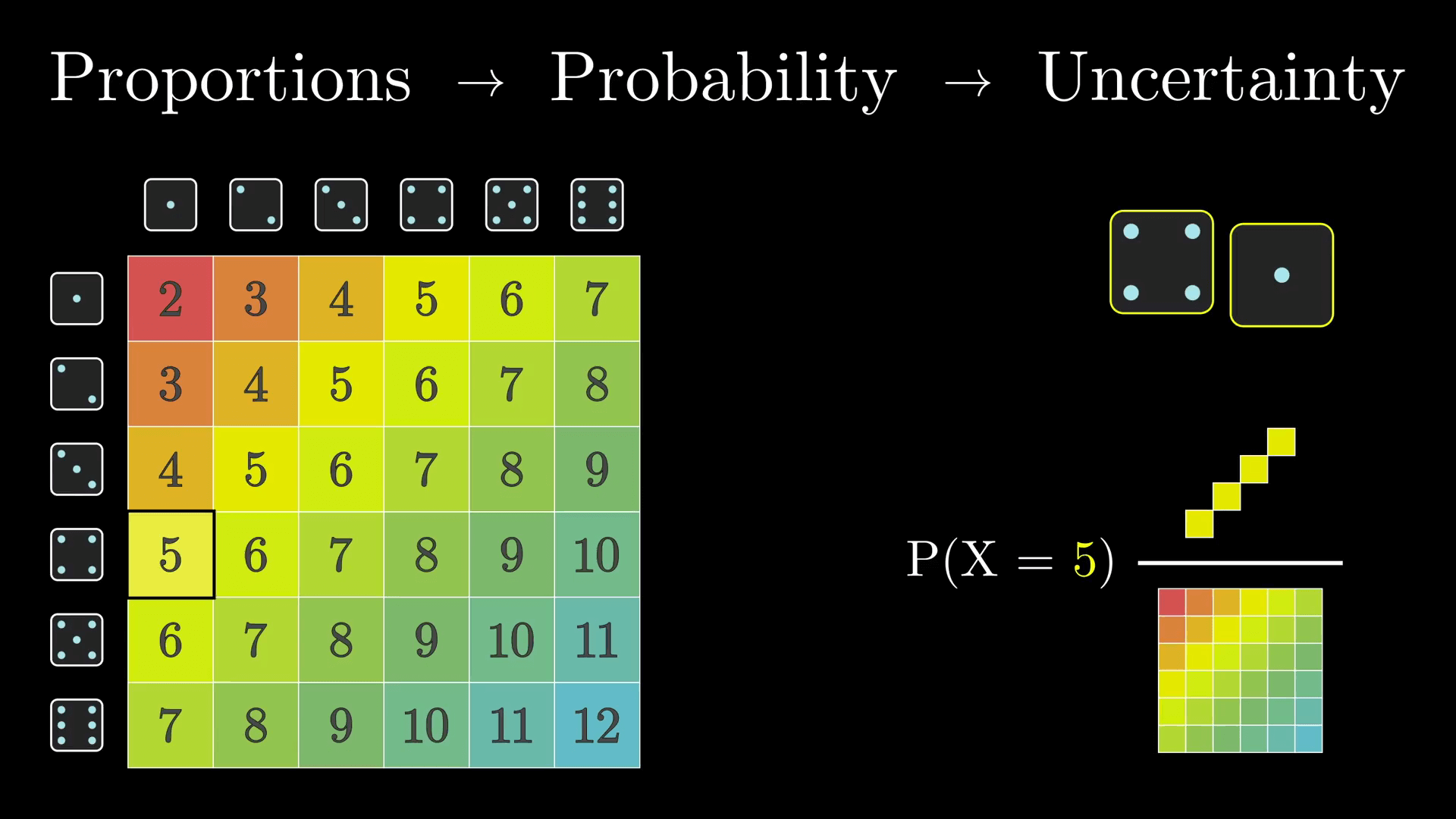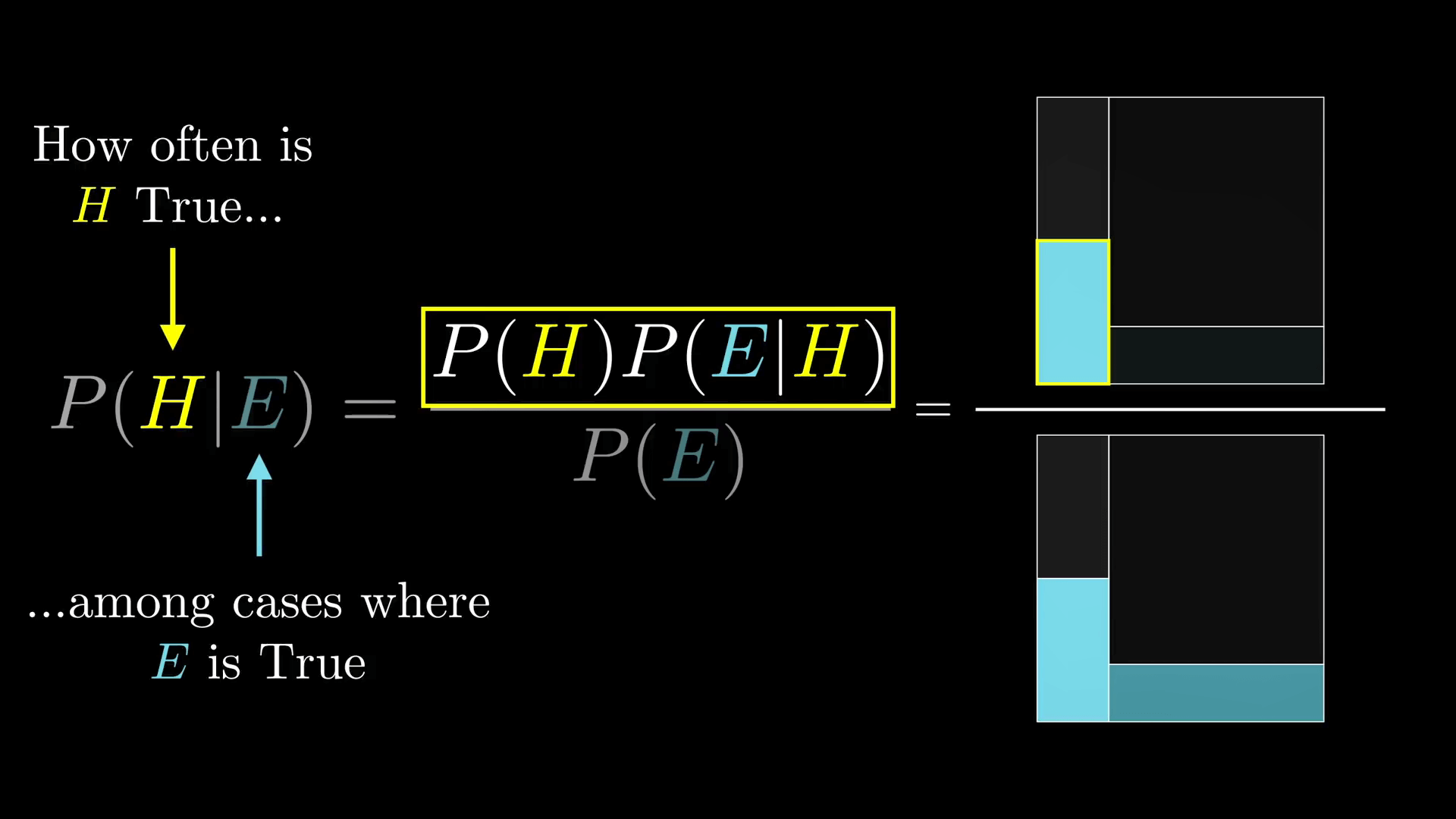## 补充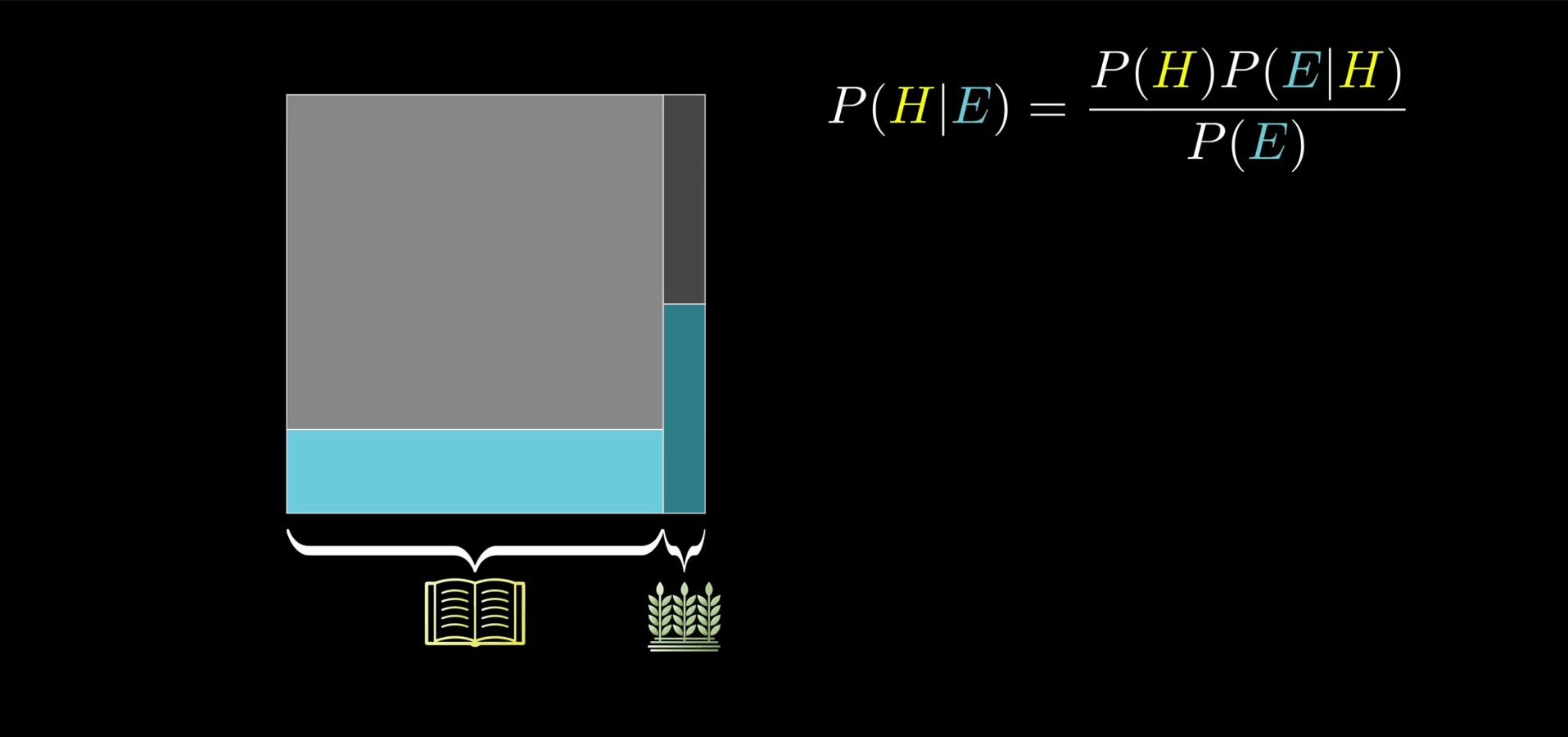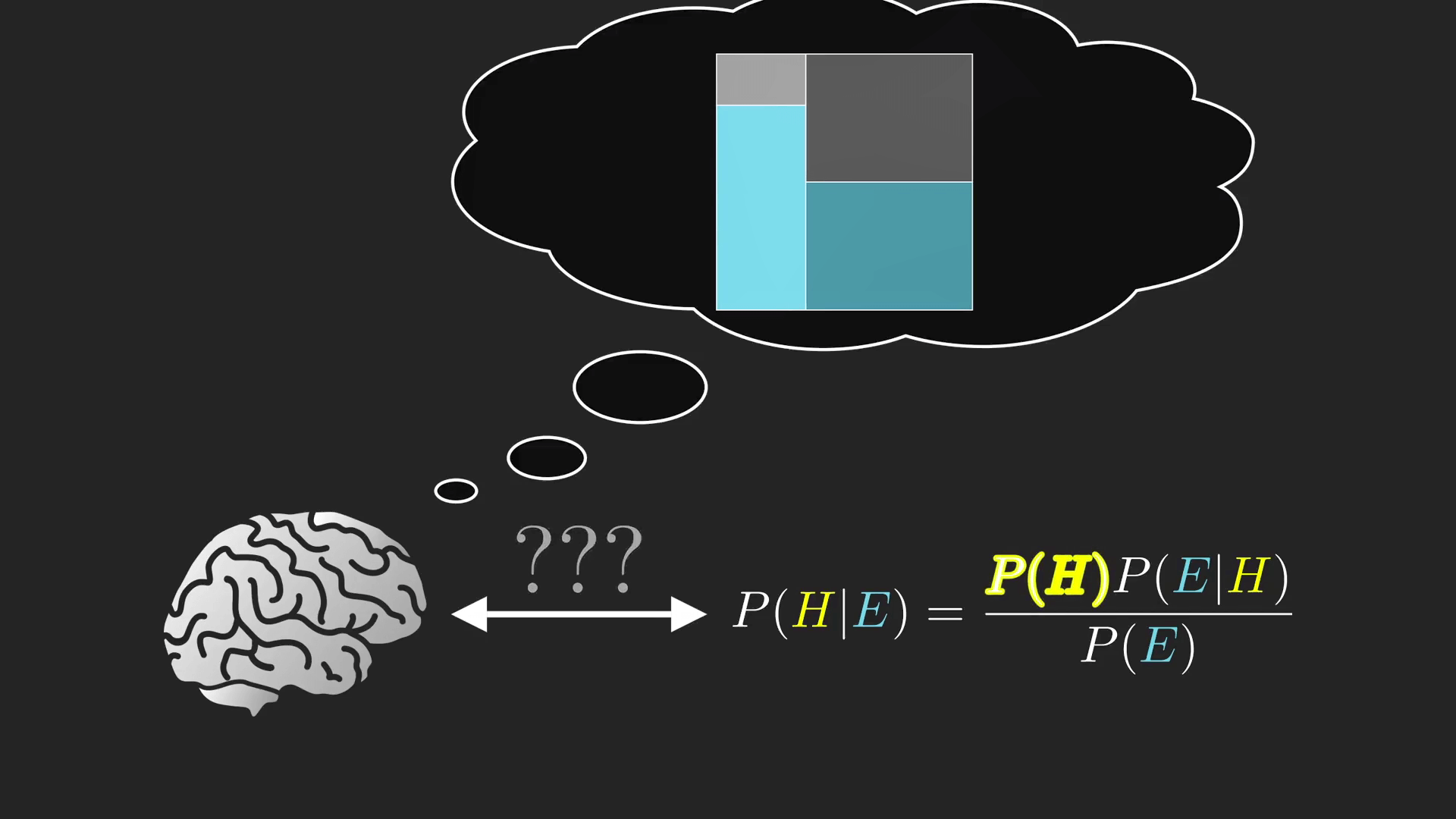• 默认
• 护眼
• 夜晚
• Serif
• Sans